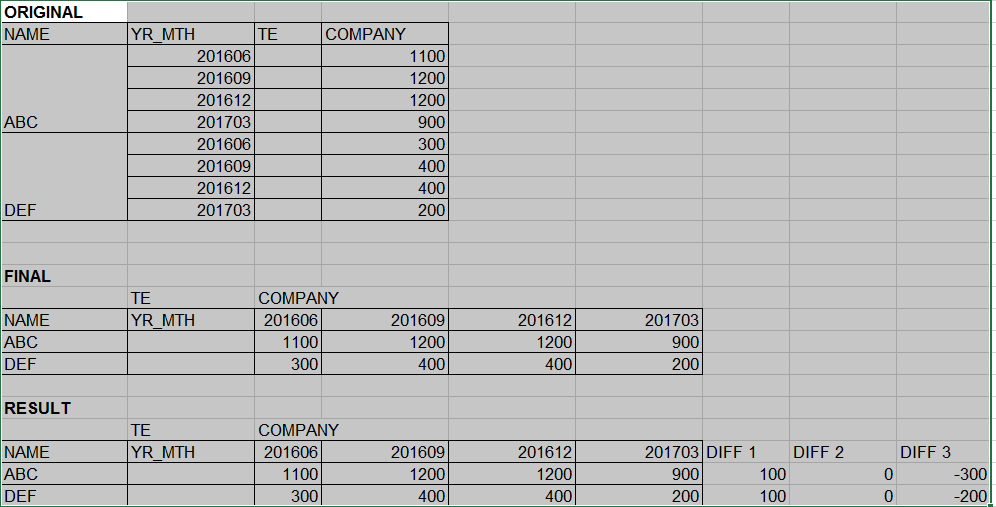# QlikView Layout & Visualizations

Discussion Board for collaboration on QlikView Layout & Visualizations.

Highlighted
Contributor

## Dynamic 4 rolling quarter

I have a Date1 column in this format - YYYYMM.

Date1 = 201703 is 1st quarter

Date1 = 201706 is 2nd quarter

Date1 = 201709 is 3rd quarter

Date1 = 201712 is 4th quarter

Date1 = 201803 is 1st quarter

Date1 = 201806 is 2nd quarter

Date1 = 201809 is 3rd quarter

Date1 = 201812 is 4th quarter

How to create a rolling 4 quarter so that in a pivot table, the 4 quarters (as 4 columns) dynamically get updated?

1 Solution

Accepted Solutions
Valued Contributor

## Re: Dynamic 4 rolling quarter

Hi,

='Q' & Right(Date1,2)/3

6 RepliesMVP

## Re: Dynamic 4 rolling quarter

Are you looking to show Max 4 quarters in your pivot table? May be this

If Date1 is in number format.....

{<Date1 = {"\$(='>' & (Max(Date1)-100) & '<=' & Max(Date1))"}>}

If Date1 is in Datef format (like you mentioned)

{<Date1 = {"\$(='>' & Date(AddYears(Max(Date1), -1), 'YYYYMM') & '<=' & Date(Max(Date1), 'YYYYMM'))"}>}

Valued Contributor

## Re: Dynamic 4 rolling quarter

Hi,

='Q' & Right(Date1,2)/3

Contributor

## Re: Dynamic 4 rolling quarter

Hi Sunny, Yes I'm looking for last 4 quarters. I've the Date as a dimension in my pivot table, not as expression. How does this fit in this case?

Dimension is:
PICK(Dim, Date1, 'DIFF1', 'DIFF2', 'DIFF3')

Expression is:

Pick(Dim,
'\$'&Num(round(Sum(COMPANY) * 1.05),'###########,###'),
Num((Sum({<YR_MTH = {
"\$(=Min(YR_MTH, 2))"}>} COMPANY) - Sum({<YR_MTH = {"\$(=Min(YR_MTH, 1))"}>} COMPANY))/100, '#.0%'),
Num((Sum({<YR_MTH = {
"\$(=Min(YR_MTH, 3))"}>} COMPANY) - Sum({<YR_MTH = {"\$(=Min(YR_MTH, 2))"}>} COMPANY))/100, '#.0%'),
Num((Sum({<YR_MTH = {
"\$(=Min(YR_MTH, 4))"}>} COMPANY) - Sum({<YR_MTH = {"\$(=Min(YR_MTH, 3))"}>} COMPANY))/100, '#.0%'))Contributor

## Re: Dynamic 4 rolling quarter

Hi Fer Fer, that gave me 3 quarters from last year but dropped the current quarter. So, for instance if 201703 is the latest date which is 1st quarter 2017, I got Q2, Q3, Q4 for 2016 only and it dropped the 201703 (Q1 for 2017).

Contributor

## Re: Dynamic 4 rolling quarter

Never mind, the Q1 didn't get dropped. It moved to the last column, so it wasn't in the same sorting order.

Contributor